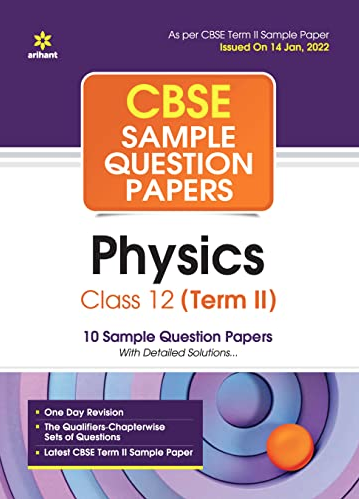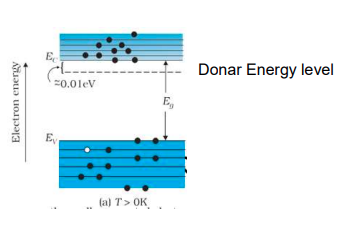# In a pure semiconductor crystal of Si, if antimony is added then what type of extrinsic semiconductor is obtained. Draw the energy band diagram of this extrinsic semiconductor so formed.

CBSE Sample Question Paper, Class 12 Physics Term 2 Question - In a pure semiconductor crystal of Si, if antimony is added then what type of extrinsic semiconductor is obtained. Draw the energy band diagram of this extrinsic semiconductor so formed.## In a pure semiconductor crystal of Si, if antimony is added then what type of extrinsic semiconductor is obtained. Draw the energy band diagram of this extrinsic semiconductor so formed.

### ANS 1 As given in the statement antimony is added to pure Si crystal, then a n -type extrinsic semiconductor would be so obtained, Since antimony(Sb) is a pentavalent impurity. Energy level diagram of n-type semiconductor